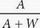# SAT Math Multiple Choice Question 182: Answer and Explanation

### Test Information

Question: 182

2. Y =A gardener prepares a mixture of fertilizer with concentration, by volume, equal to Y. It is prepared by mixing a volume of fertilizer given by A with a volume of water given by W. The expression above represents the mixture described. What physical quantity does the term A + W represent in the equation above?

• A. The volume of the mixture
• B. The mass of fertilizer added
• C. The volume of the fertilizer in the mixture
• D. The concentration of the fertilizer